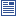Accessibility options:

# Probability resources

Show me all resources applicable to

### 04. Tests and Quizzes (5)Business Statistics - Numbas
6 questions on standard statistical distributions. Binomial, Poisson, Normal, Uniform, Exponential. Numbas resources have been made available under a Creative Commons licence by Bill Foster and Christain Perfect, School of Mathematics & Statistics at Newcastle University.Business Statistics 2 - Numbas
5 questions. 1. Counting number of occurences of an event in a sample space with given size and finding the probability of the event. 2. Finding probabilities from a survey given a table of data. 3. Example showing how to calculate the probability of A or B using the law p(A or B)=p(A)+p(B)-p(A and B). Also converting percentages to probabilities. 4. Choose whether given three given pairs of events are independent or not. 5. Given data on probabilities of three levels of success of three options and projections of the profits that the options will accrue depending on the level of success, find the expected monetary value (EMV) for each option and choose the one with the greatest EMV. Numbas resources have been made available under a Creative Commons licence by the School of Mathematics & Statistics at Newcastle University.Business Statistics 3 - Numbas
. 1. Given descriptions of 3 random variables, decide whether or not each is from a Poisson or Binomial distribution. 2. Application of the Poisson distribution given expected number of events per interval. Finding probabilities using the Poisson distribution. 3. Application of the binomial distribution given probabilities of success of an event. Finding probabilities using the binomial distribution. 4. Given a random normal variable X as N(m, var) find P(X > a),a > m; P(X < b), b < m. 5. Given uniform distribution X, calculating the expectation and variance. Also finding P(X=a) for a given value a.6. Question on the exponential distribution involving a time intervals and arrivals application, finding expectation and variance. Also finding the probability that a time interval between arrivals is less than a given period. All parameters and times randomised. Numbas resources have been made available under a Creative Commons licence by the School of Mathematics & Statistics at Newcastle University.Probability distributions - Numbas
6 Numbas questions on standard statistical distributions; Binomial, Poisson, Normal, Uniform, Exponential. Numbas resources have been made available under a Creative Commons licence by Bill Foster and Christain Perfect, School of Mathematics & Statistics at Newcastle University.Probability mass functions - Numbas
Questions on using a probability mass function; determining if a given function is a probability mass function and finding and using the probability mass function of a discrete distribution. Numbas resources have been made available under a Creative Commons licence by Bill Foster and Christain Perfect, School of Mathematics & Statistics at Newcastle University.

### 07. Community Project (1)6 Probability (Workshop SOURCE)
This PowerPoint workshop provides a presentation on basic probability theory. This resource has been contributed to the statstutor Community Project by Peter Samuels, Birmingham City University under a Creative Commons licence CC-BY-SA and reviewed by Ellen Marshall, University of Sheffield. The zip file contains the source file and the associated statstutor metadata spreadsheet.

### 08. Staff Resources (1)Introductory Statistics and Hypothesis Testing (PowerPoint Workshop)
These slides are aimed to be used in a workshop to train mathematics (or new statistics) tutors who need to provide statistics support. They cover key topics including hypothesis testing and choosing the right test. These slides were developed and contributed to the statstutor Community Project by Alun Owen (University of Worcester) and Ellen Marshall (University of Sheffield) and reviewed by Ruth Fairclough (University of Wolverhampton).

### 10. Workshops (1)6 Probability (Workshop)
This PowerPoint workshop provides a presentation on basic probability theory. This resource has been contributed to the statstutor Community Project by Peter Samuels, Birmingham City University and reviewed by Ellen Marshall, University of Sheffield.